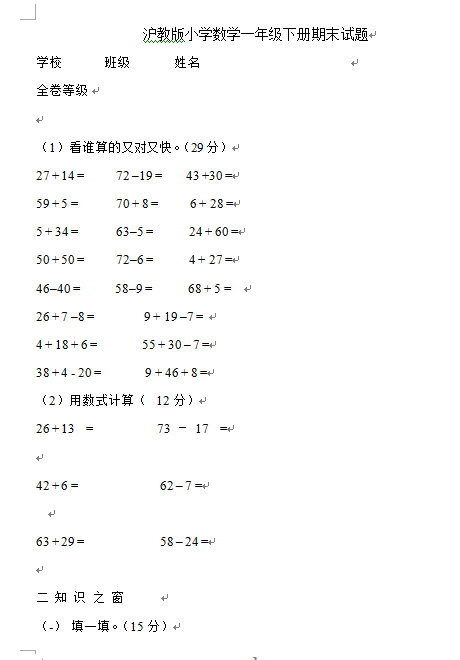（1）看谁算的又对又快。（29分）

27 + 14 =     72 –19 =    43 +30 =

59 + 5 =      70 + 8 =     6 + 28 =

5 + 34 =      63–5 =      24 + 60 =

【DOC文档4页】小学沪教版数学一年级下册期末试题A4电子版资料_可直接打印_会员免费下载##### 评论信息